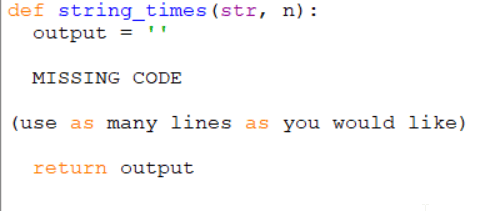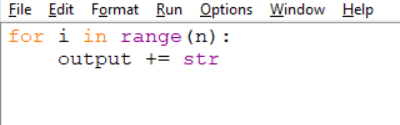Find the Error
Python History
Variables and Lists
Output
Loops, Loops, Loops

### 100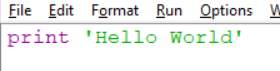The print function is missing parentheses.

### 100

Python is a:

A) Snake hisss hisss

B) Programming language

C) Traffic Cone

B) Programming language.

### 100

What kind of variables is var?

var = 12.2

Float

### 100

What is the output of the following: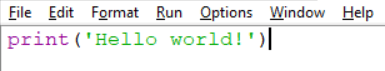Hello world!

### 100

What are the two basic kinds of loops?

For loops and while loops.

### 200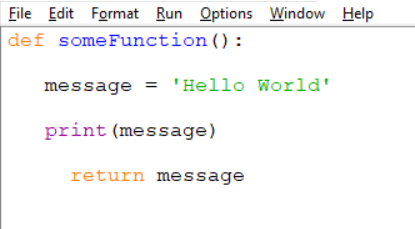Return is indented too much.

### 200

Python is:

A) Dynamically Typed

B) Statically Typed

C) Not Typed

A) Dynamically Typed

### 200

Name 3 types of variables in Python.

int, long, float, complex, String, boolean

### 200

What is the output of the following:1

### 200

When should you use a for loop and when should you use a while loop?

For loop: When you want to repeat a section of code a specific number of times.

While loop: When you do not know how many times you want to repeat a section of code.

### 300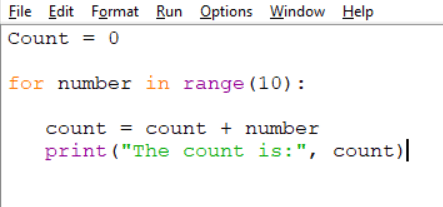The variable "count" does not exist. Capitalization matters!

### 300

When was Python first released?

A) 1960's

B) 1970's

C) 1990's

D) 2000's

C) 1990's

### 300

What kind of variables is var?

var = '12'

String

### 300

Which one of the following happens first?
a) Exponents
c) Multiplication
d) Parentheses

Parentheses

### 300

What is the output of the following: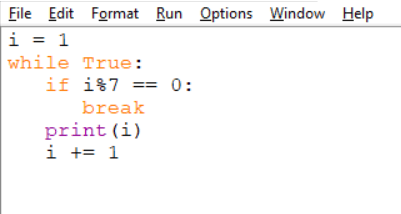1 2 3 4 5 6

### 400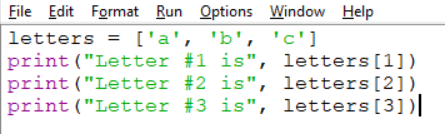letters does not exist. Indices start at 0, not 1.

### 400

Name one of Python's core design philosophies.

• Beautiful is better than ugly
• Explicit is better than implicit
• Simple is better than complex
• Complex is better than complicated

### 400

What is the proper syntax to add the number 5 to the following list?

nums = [1, 2, 3, 4]

nums = nums + 

### 400

What is the output of the following?Error. Needs to have a negative count.

### 400

What are x, y, and z in the loop below if we want it to count from 50 down to 10 by 4s?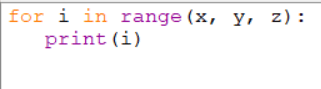x = 50

y = 9 (can also be 6, 7, or 8)

z = -4

### 500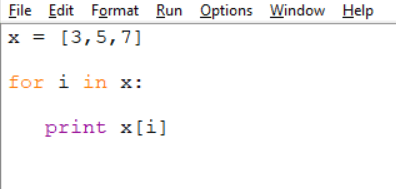i will equal 3 then 5 then 7. i does not have enough values for i, i, or i to be defined, so we get an error.

We should instead use for i in range(len(x)):

### 500

What is Python named after?

Monty Python, the British comedy group.

### 500

Fill in the line of code below to complete a function that returns true if the list ends with a 6: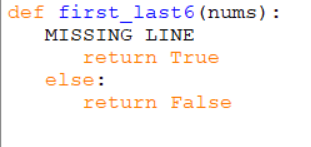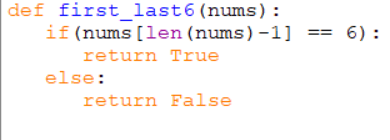### 500

What is the output of the following?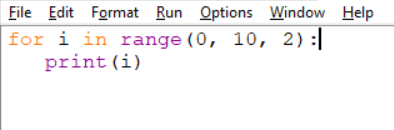0

2

4

6

8

### 500

Given a string and an int n return a larger string that is n copies of the original string.

string_times('Hi', 2) → 'HiHi'

string_times('Hi', 3) → 'HiHiHi'

Fill in the missing code: